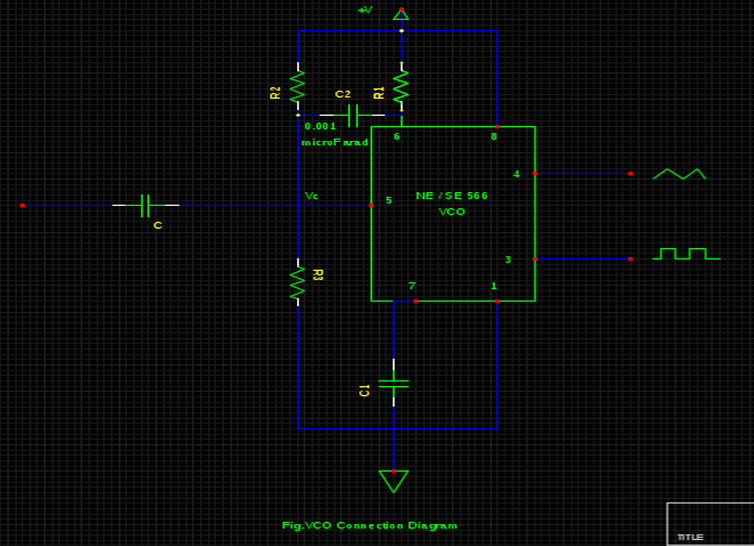# Numerical on VCO Connection.

## For the circuit shown below, +V= 15 V, R2 = 1K, R1 = R3 = 8K & C1 = 0.001 μF. Compute (a) nominal frequency of output waveforms & (b) Modulation in the output frequencies if Vc changes in the mist of 10 V to 11.5V.a. 26.17 & 33.34 kHz
b. 35.60 kHz & 10 kHz
c. 30.47 kHz & 10 kHz
d. 22.56 & 33.34 kHz

Correct Answer : c. 30.47 kHz & 10 kHz

Explanation :

(a) By applying voltage -divider rule, initial voltage at terminal 5 is given by,

Vc = (20 K) (15) / 11.5 K = 26.09 V
Thus, the frequency of output waveform (nominal frequency) is approximated by,

fo ≈ 2(+V- Vc) / R1C1 (+V)
= 2 (15 – 10.43) / (20K x 10-9) x (15)
= 30.47 kHz

(b) Thus, the modulation in the output frequencies can be calculated by initially substituting Vc = 10 V & then 11.5 V

fo = 2 ( 15 – 10) / 20K x 10-9 x 15)
= 33.34 kHz

fo = 2 ( 15 – 11.5) / 20K x 10-9 x 15)
= 23.34 kHz

Hence, the variation in an output frequency can be evaluated by the difference between these both frequencies.
i.e. 33.34 – 23.34 = 10 kHz.